# JP5109027B2 - Blood vessel state evaluation apparatus, blood vessel state evaluation method, and blood vessel state evaluation program - Google Patents

Blood vessel state evaluation apparatus, blood vessel state evaluation method, and blood vessel state evaluation program Download PDF

## Info

Publication number
JP5109027B2
JP5109027B2 JP2007092522A JP2007092522A JP5109027B2 JP 5109027 B2 JP5109027 B2 JP 5109027B2 JP 2007092522 A JP2007092522 A JP 2007092522A JP 2007092522 A JP2007092522 A JP 2007092522A JP 5109027 B2 JP5109027 B2 JP 5109027B2
Authority
JP
Japan
Prior art keywords
blood vessel
transfer function
phase difference
circulatory
state evaluation
Prior art date
Legal status (The legal status is an assumption and is not a legal conclusion. Google has not performed a legal analysis and makes no representation as to the accuracy of the status listed.)
Active
Application number
JP2007092522A
Other languages
Japanese (ja)
Other versions
JP2008246010A5 (en
JP2008246010A (en
Inventor

Original Assignee

オムロンヘルスケア株式会社
Priority date (The priority date is an assumption and is not a legal conclusion. Google has not performed a legal analysis and makes no representation as to the accuracy of the date listed.)
Filing date
Publication date
Application filed by 国立大学法人京都大学, オムロンヘルスケア株式会社 filed Critical 国立大学法人京都大学
Priority to JP2007092522A priority Critical patent/JP5109027B2/en
Publication of JP2008246010A publication Critical patent/JP2008246010A/en
Publication of JP2008246010A5 publication Critical patent/JP2008246010A5/ja
Application granted granted Critical
Publication of JP5109027B2 publication Critical patent/JP5109027B2/en
Active legal-status Critical Current
Anticipated expiration legal-status Critical

• 210000004204 Blood Vessels Anatomy 0.000 title claims description 174
• 238000004364 calculation methods Methods 0.000 claims description 64
• 230000000875 corresponding Effects 0.000 claims description 45
• 230000002093 peripheral Effects 0.000 claims description 24
• 230000002792 vascular Effects 0.000 claims description 24
• 238000006243 chemical reactions Methods 0.000 claims description 11
• 238000003860 storage Methods 0.000 claims description 9
• 230000017531 blood circulation Effects 0.000 claims description 6
• 230000036772 blood pressure Effects 0.000 claims description 5
• 230000004087 circulation Effects 0.000 claims description 2
• 238000010586 diagrams Methods 0.000 description 32
• 239000011133 lead Substances 0.000 description 27
• 210000001367 Arteries Anatomy 0.000 description 26
• 238000000034 methods Methods 0.000 description 25
• 0 *C1C23C(I)(*(C(/N=C/[Am])(*)[C@@H]([C@@H]([C@H]1C)*)C)C3C)C2 Chemical compound *C1C23C(I)(*(C(/N=C/[Am])(*)[C@@H]([C@@H]([C@H]1C)*)C)C3C)C2 0.000 description 22
• 210000002565 Arterioles Anatomy 0.000 description 20
• 238000010587 phase diagrams Methods 0.000 description 18
• 206010003210 Arteriosclerosis Diseases 0.000 description 16
• 210000004369 Blood Anatomy 0.000 description 11
• 239000008280 blood Substances 0.000 description 11
• 238000009826 distribution Methods 0.000 description 10
• 230000014509 gene expression Effects 0.000 description 8
• 210000003423 Ankle Anatomy 0.000 description 6
• 239000011159 matrix materials Substances 0.000 description 6
• 210000001737 Ankle Joint Anatomy 0.000 description 5
• 239000011886 peripheral blood Substances 0.000 description 5
• 238000002310 reflectometry Methods 0.000 description 5
• 230000004044 response Effects 0.000 description 5
• 230000001902 propagating Effects 0.000 description 4
• 230000001105 regulatory Effects 0.000 description 4
• 210000000709 Aorta Anatomy 0.000 description 3
• 239000003570 air Substances 0.000 description 3
• 230000005284 excitation Effects 0.000 description 3
• 210000001736 Capillaries Anatomy 0.000 description 2
• 230000002238 attenuated Effects 0.000 description 2
• 235000020127 ayran Nutrition 0.000 description 2
• 238000003825 pressing Methods 0.000 description 2
• OPTLFHZXCSPZDJ-IDKOKCKLSA-N C(C)C12CCCC(C1)[C@@H]2C Chemical compound C(C)C12CCCC(C1)[C@@H]2C OPTLFHZXCSPZDJ-IDKOKCKLSA-N 0.000 description 1
• 208000008787 Cardiovascular Diseases Diseases 0.000 description 1
• 230000036536 Cave Effects 0.000 description 1
• 210000002321 Radial Artery Anatomy 0.000 description 1
• 280000638271 Reference Point companies 0.000 description 1
• 238000004458 analytical methods Methods 0.000 description 1
• 230000006399 behavior Effects 0.000 description 1
• 238000009530 blood pressure measurement Methods 0.000 description 1
• 239000003990 capacitor Substances 0.000 description 1
• 238000005516 engineering processes Methods 0.000 description 1
• 238000007689 inspection Methods 0.000 description 1
• 239000004973 liquid crystal related substances Substances 0.000 description 1
• 230000004048 modification Effects 0.000 description 1
• 238000006011 modification reactions Methods 0.000 description 1
• 229910021421 monocrystalline silicon Inorganic materials 0.000 description 1
• 238000005457 optimization Methods 0.000 description 1
• 230000035479 physiological effects, processes and functions Effects 0.000 description 1
• 238000005070 sampling Methods 0.000 description 1
• 239000004065 semiconductors Substances 0.000 description 1
• 230000001360 synchronised Effects 0.000 description 1
• 230000002123 temporal effects Effects 0.000 description 1
• 210000001519 tissues Anatomy 0.000 description 1

## Images

•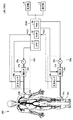••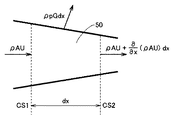•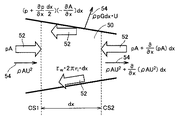•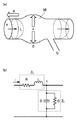•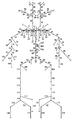•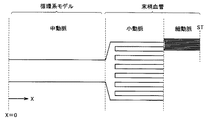•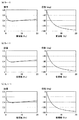•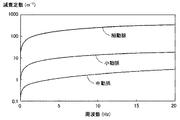•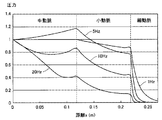•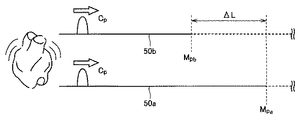•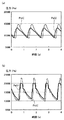•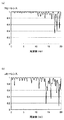•••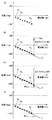••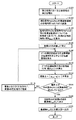•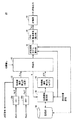••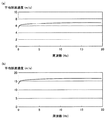•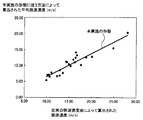•## Classifications

• AHUMAN NECESSITIES
• A61MEDICAL OR VETERINARY SCIENCE; HYGIENE
• A61BDIAGNOSIS; SURGERY; IDENTIFICATION
• A61B5/00Detecting, measuring or recording for diagnostic purposes; Identification of persons
• A61B5/02Detecting, measuring or recording pulse, heart rate, blood pressure or blood flow; Combined pulse/heart-rate/blood pressure determination; Evaluating a cardiovascular condition not otherwise provided for, e.g. using combinations of techniques provided for in this group with electrocardiography or electroauscultation; Heart catheters for measuring blood pressure
• A61B5/026Measuring blood flow
• A61B5/0285Measuring or recording phase velocity of blood waves
• AHUMAN NECESSITIES
• A61MEDICAL OR VETERINARY SCIENCE; HYGIENE
• A61BDIAGNOSIS; SURGERY; IDENTIFICATION
• A61B5/00Detecting, measuring or recording for diagnostic purposes; Identification of persons
• A61B5/02Detecting, measuring or recording pulse, heart rate, blood pressure or blood flow; Combined pulse/heart-rate/blood pressure determination; Evaluating a cardiovascular condition not otherwise provided for, e.g. using combinations of techniques provided for in this group with electrocardiography or electroauscultation; Heart catheters for measuring blood pressure
• A61B5/02007Evaluating blood vessel condition, e.g. elasticity, compliance
• AHUMAN NECESSITIES
• A61MEDICAL OR VETERINARY SCIENCE; HYGIENE
• A61BDIAGNOSIS; SURGERY; IDENTIFICATION
• A61B5/00Detecting, measuring or recording for diagnostic purposes; Identification of persons
• A61B5/68Arrangements of detecting, measuring or recording means, e.g. sensors, in relation to patient
• A61B5/6801Arrangements of detecting, measuring or recording means, e.g. sensors, in relation to patient specially adapted to be attached to or worn on the body surface
• A61B5/6813Specially adapted to be attached to a specific body part
• A61B5/6828Leg
• AHUMAN NECESSITIES
• A61MEDICAL OR VETERINARY SCIENCE; HYGIENE
• A61BDIAGNOSIS; SURGERY; IDENTIFICATION
• A61B5/00Detecting, measuring or recording for diagnostic purposes; Identification of persons
• A61B5/68Arrangements of detecting, measuring or recording means, e.g. sensors, in relation to patient
• A61B5/6801Arrangements of detecting, measuring or recording means, e.g. sensors, in relation to patient specially adapted to be attached to or worn on the body surface
• A61B5/6813Specially adapted to be attached to a specific body part
• A61B5/6824Arm or wrist

## Description

The present invention relates to a blood vessel state evaluation apparatus, a blood vessel state evaluation method, and a blood vessel state evaluation program for evaluating the state of a blood vessel constituting a living body. In particular, the degree of arteriosclerosis of a blood vessel is evaluated after modeling a blood vessel path as a transfer function. It is related to the technology.

In recent years, cardiovascular diseases caused by arteriosclerosis have increased, and in response to this, an evaluation apparatus for evaluating the degree of arteriosclerosis of blood vessels has been put into practical use. A pulse wave velocity method is known as a representative method for evaluating the degree of arteriosclerosis. This pulse wave velocity method utilizes the fact that there is a correlation between the velocity (pulse wave velocity) at which a blood pressure change accompanying the heartbeat propagates on the blood vessel and the elasticity (rigidity) of the blood vessel. That is, when the pulse wave travels through the blood vessel, which is an elastic tube, the pulse wave velocity increases as the tube wall becomes harder, the inner diameter is thinner, and the tube thickness is thicker. You can know the degree. In particular, an evaluation apparatus based on the baPWV method (brachial-ankle Pulse Wave Velocity method) using time waveforms of blood pressure in both upper arms and both ankles has been put into practical use.

As a method for measuring such a pulse wave velocity, for example, Japanese Patent Laying-Open No. 2006-326334 (Patent Document 1) discloses a temporal relationship between voltage waveforms obtained from adjacent voltage electrode pairs among a plurality of voltage waveforms. A pulse wave propagation speed or pulse wave propagation time between adjacent voltage electrodes using a detecting means for detecting a distortion and a distance and / or a time lag between adjacent voltage electrodes for all of a plurality of voltage waveforms. There is disclosed a pulse wave velocity measuring device having a calculating means for obtaining the rate of change of the pulse wave.
JP 2006-326334 A

As described in Japanese Patent Laid-Open No. 2006-326334 (Patent Document 1), as a method for measuring a pulse wave velocity, a time difference (delay time) between time waveforms measured at a plurality of points on a blood vessel path is obtained. The pulse wave velocity is calculated by dividing the path difference from the heart at each point by the time difference.

However, since the actual pulse wave velocity depends on the propagation path and frequency, the exact pulse wave velocity cannot be calculated simply by dividing the path difference by the time difference. That is, a value deviating from the original pulse wave velocity may be calculated depending on the blood vessel diameter and blood vessel length of the person to be evaluated, the frequency component included in the pulse wave, and the like. For this reason, there has been a problem that the evaluation accuracy of the degree of arteriosclerosis cannot be increased so much.

Accordingly, the present invention has been made to solve such a problem, and an object of the present invention is to provide a blood vessel state evaluation apparatus, a blood vessel state evaluation method, and a blood vessel state capable of evaluating the degree of arteriosclerosis with higher accuracy. To provide an evaluation program.

A blood vessel state evaluation device according to an aspect of the present invention includes storage means for storing a circulatory system model obtained by dividing a blood vessel constituting a living body into a plurality of sections, and the circulatory system model stores each of the plurality of sections. Includes representative shape values. Furthermore, the blood vessel state evaluation apparatus is attached to the first measurement part of the living body and measures the time waveform of the first biological signal, and is attached to the second measurement part of the living body and is synchronized with the first measurement part. Then, based on the second measuring means for measuring the time waveform of the second biological signal and the phase difference for each frequency component between the first biological signal and the second biological signal, the actually measured phase difference characteristic is calculated. Based on the first calculation means, the first transfer function defined based on the circulatory system model corresponding to the vascular route to the first measurement site, and based on the circulatory system model corresponding to the vascular route to the second measurement site And a second calculating means for calculating a phase difference characteristic between the second transfer function and the second transfer function, wherein the first transfer function and the second transfer function include an elasticity variable indicating a degree of elasticity of the blood vessel. Further, the blood vessel state evaluation apparatus is a search unit that determines the elasticity variable by fitting the phase difference characteristic calculated by the second calculation unit based on the actually measured phase difference characteristic calculated by the first calculation unit. Is provided.

According to the present invention, based on the circulatory system model, the first and second transfer functions are defined corresponding to the blood vessel paths to the first and second measurement sites, respectively, and then the first transfer function and the second transfer function are defined. A phase difference characteristic between the transfer function and the transfer function is calculated. Here, the first and second transfer functions include an elasticity variable indicating the elasticity of the blood vessel. On the other hand, after the time waveforms of the first and second biological signals are measured from the first and second measurement sites, respectively, the phase difference for each frequency component between the first biological signal and the second biological signal is calculated. Based on this, an actually measured phase difference characteristic is calculated. Further, the elasticity variable is determined by fitting the phase difference characteristic between the first transfer function and the second transfer function based on the actually measured phase difference characteristic.

Here, since the first and second transfer functions are defined including the frequency characteristic of the pulse wave, the optimum solution of the elasticity variable reflecting the frequency characteristic of the actually measured signal is obtained by fitting. . Therefore, the blood vessel state (degree of arteriosclerosis) can be evaluated with higher accuracy in consideration of the frequency characteristics.

Preferably, the blood vessel state evaluation apparatus further includes transfer function calculating means for calculating the first and second transfer functions based on the shape values of the sections corresponding to the blood vessel paths to the first and second measurement sites, respectively. Prepare.

Preferably, the transfer function calculating means calculates the first and second transfer functions using the distribution constant model associated with each section and having the blood vessel pressure and the blood flow rate as input variables. Each includes a longitudinal impedance corresponding to the ease of blood flow in a corresponding section and a lateral impedance having an elasticity variable.

Preferably, the blood vessel state evaluation device further includes a pulse wave velocity calculating unit that calculates a pulse wave velocity in the blood vessel based on the elasticity variable fitted by the searching unit.

More preferably, the pulse wave velocity calculating means calculates the pulse wave velocity based on the shape value of each section corresponding to the blood vessel path to the first measurement site and the shape value of each section corresponding to the blood vessel route to the second measurement site. Is calculated.

Preferably, the circulatory system model includes a blood vessel diameter and a blood vessel length as shape values.
Preferably, the circulatory system model is obtained by classifying blood vessels constituting a living body into a plurality of sections and modeling blood vessels belonging to at least one of the plurality of sections.

More preferably, the blood vessels constituting the living body are classified into a plurality of sections based on the size of the blood vessel diameter.

Preferably, the transfer function calculating means adds a peripheral part model obtained by modeling a blood vessel not modeled in the circulatory system model among the blood vessels included in each section to the circulatory system model corresponding to each section. Then, the transfer function is calculated.

More preferably, the transfer function calculating means calculates the peripheral part model of the section by converting the circulatory system model of each section based on the blood vessel shape difference.

More preferably, the transfer function calculating means calculates the transfer function under the non-reflection condition at the end of the peripheral part model.

Preferably, the blood vessel state evaluation device includes a first frequency converting unit that calculates a first phase characteristic indicating a phase for each frequency component from the first biological signal, and a first frequency indicating the phase for each frequency component from the second biological signal. And a second frequency conversion unit that calculates a two-phase characteristic, and the first calculation unit calculates the difference phase data by subtracting the first phase characteristic and the second phase characteristic . Furthermore, the blood vessel state evaluation apparatus calculates the actually measured phase difference characteristic by correcting the phase shift caused by the period delay in the difference phase data in units of phases corresponding to one or two or more periods.

Preferably, the first calculation means calculates the actually measured phase difference characteristic using a frequency component in which the coherence value between the first biological signal and the second biological signal is higher than a predetermined threshold value.

According to another aspect of the present invention, using a circulatory system model obtained by dividing a blood vessel constituting a living body into a plurality of sections, a method for determining an elasticity variable indicating a degree of elasticity of a blood vessel , The circulatory system model includes shape values representing each of the plurality of sections. The method measures the time waveform of the first biological signal from the first measurement site of the living body, measures the time waveform of the second biological signal from the second measurement site of the living body, the first biological signal, and the second A step of calculating an actually measured phase difference characteristic based on a phase difference for each frequency component between the biological signal and a first defined based on a circulatory system model corresponding to a blood vessel path to the first measurement site Calculating a phase difference characteristic between one transfer function and a second transfer function defined based on a circulatory system model corresponding to a blood vessel path to a second measurement site, and the first transfer function and the second transfer function includes a bullet Chikarado variable. Furthermore, the how, based on the phase difference characteristics of the actual measurement, by fitting the phase difference characteristics between the first transfer function and the second transfer function, comprising the step of determining the elasticity variable.

According to still another aspect of the present invention, a computer having an arithmetic processing unit uses a circulatory system model in which a blood vessel constituting a living body is divided into a plurality of sections to model the state of blood vessels constituting the living body. The circulatory system model includes shape values representing each of a plurality of sections. In the blood vessel state evaluation program, the arithmetic processing unit acquires the time waveform of the first biological signal measured at the first measurement site of the living body and the time waveform of the second biological signal measured at the second measurement site of the living body. Obtaining a measured phase difference characteristic based on the phase difference of each frequency component between the first biological signal and the second biological signal, and the arithmetic processing unit The first transfer function defined based on the circulatory system model corresponding to the vascular route to the first measurement site and the first transfer function defined based on the circulatory system model corresponding to the vascular route to the second measurement site Calculating a phase difference characteristic between the two transfer functions, and the first transfer function and the second transfer function include a elasticity variable indicating a degree of elasticity of the blood vessel. Furthermore, the blood vessel state evaluation program has an elasticity that is obtained when the arithmetic processing unit fits the phase difference characteristic between the first transfer function and the second transfer function based on the actually measured phase difference characteristic. Determining a degree variable.

According to the present invention, a blood vessel state evaluation apparatus, a blood vessel state evaluation method, and a blood vessel state evaluation program that can evaluate the degree of arteriosclerosis with higher accuracy can be realized.

Embodiments of the present invention will be described in detail with reference to the drawings. Note that the same or corresponding parts in the drawings are denoted by the same reference numerals and description thereof will not be repeated.

[Embodiment 1]
(Device configuration)
FIG. 1 is a schematic configuration diagram of a blood vessel state evaluation apparatus 100 according to Embodiment 1 of the present invention.

Referring to FIG. 1, blood vessel state evaluation apparatus (hereinafter also simply referred to as “evaluation apparatus”) 100 according to Embodiment 1 of the present invention includes a control unit 2, a display unit 4, an operation unit 6, and a measurement unit. 20a, 20b.

The control unit 2 is a device that controls the entire evaluation apparatus 100, and typically includes a CPU (Central Processing Unit) 10, a ROM (Read Only Memory) 12, and a RAM (Random Access Memory) 14. Consists of.

The CPU 10 corresponds to an arithmetic processing unit, reads a program stored in advance in the ROM 12, and executes the program while using the RAM 14 as a work memory. The ROM 12 stores at least a circulatory system model, which will be described later, in advance, and the CPU 10 refers to the circulatory system model when executing the program storing the blood vessel state evaluation method according to the present embodiment.

In addition, a display unit 4 and an operation unit 6 are connected to the control unit 2. The display unit 4 prompts the user to input various settings, and displays the calculation results from the control unit 2. On the other hand, the user operates the operation unit 6 while confirming the content displayed on the display unit 4 to perform a desired setting input or operation. In addition, the display part 4 consists of LED (Light Emitting Diode), LCD (Liquid Crystal Display) etc. as an example.

More specifically, the control unit 2 gives measurement commands to the measurement units 20a and 20b and receives measurement signals Pa (t) and Pb (t) measured in response to the measurement commands. Based on the measurement signals Pa (t) and Pb (t), the blood vessel state evaluation method according to the present embodiment is executed.

The measurement units 20a and 20b pressurize internal pressures (hereinafter referred to as “cuff pressure”) of the press cuffs (air bags) 24a and 24b attached to predetermined measurement sites of the subject 200, and biological signals at the respective measurement sites. A time waveform of a pulse wave (as an example) is measured. As will be described later, the control unit 2 calculates the actually measured phase difference characteristic based on the phase difference for each frequency component between the measurement signal Pa (t) and the measurement signal Pb (t). A measurement command is given simultaneously from the control unit 2 so that the measurement units 20a and 20b can measure a biological signal in synchronization with each other.

More specifically, for example, the press cuffs 24a and 24b are attached to the ankle part and the upper arm part of the subject 200, respectively, and pressurized by the air supplied from the measurement parts 20a and 20b via the pipes 22a and 22b, respectively. . By this pressurization, the press cuffs 24a and 24b are pressed to the corresponding measurement site, and the pressure change corresponding to the pulse wave of the measurement site is transmitted to the measurement units 20a and 20b via the pipes 22a and 22b, respectively. . The measurement units 20a and 20b measure the time waveform of the pulse wave at the measurement site by detecting the transmitted pressure change. Since it is preferable to perform arithmetic processing on predetermined frequency components (for example, 0 to 20 [Hz]) of the measurement signals Pa (t) and Pb (t), the measurement signals Pa (t) and Pb ( The measurement period (sampling period) of t) is preferably shorter than the time interval (for example, 25 msec) corresponding to this frequency component.

In order to perform such a measurement operation, the measurement unit 20a includes a pressure sensor 28a, a pressure regulating valve 26a, a pressure pump 25a, and a pipe 27a. The pressure sensor 28a is a detection part for detecting a pressure fluctuation transmitted through the pipe 22a, and includes, as an example, a plurality of sensor elements arranged at a predetermined interval on a semiconductor chip made of single crystal silicon or the like. The pressure regulating valve 26a is inserted between the pressure pump 25a and the pressure cuff 24a, and maintains the pressure used for pressurizing the pressure cuff 24a within a predetermined range during measurement. The pressure pump 25a operates according to a measurement command from the control unit 2 and supplies pressurized air for pressurizing the pressing cuff 24a.

Similarly, the measurement unit 20b includes a pressure sensor 28b, a pressure regulating valve 26b, a pressure pump 25b, and a pipe 27b. Since the configuration of each unit is the same as that of measurement unit 20a, detailed description will not be repeated.

In the present embodiment, as an example of a biological signal, a configuration in which a pressure change caused by a pulse wave is measured using a pressure cuff will be described. For example, a small constant current is passed through the measurement site of the subject 200. A voltage change caused by a change in impedance (biological impedance) generated according to the propagation of the pulse wave may be measured.

As for the correspondence between the evaluation apparatus 100 shown in FIG. 1 and the present invention, the measurement units 20a and 20b, the pipes 22a and 22b, and the press cuffs 24a and 24b are “first and second measurement means”. Equivalent to.

(Function block diagram)
Based on the circulatory system model stored in advance, the control unit 2 calculates two transfer functions defined corresponding to the blood vessel path to the measurement site where the pressing cuffs 24a and 24b are respectively attached. Here, each transfer function includes an elasticity variable indicating the elasticity of the blood vessel. That is, the elasticity variable is an index indicating the degree of arteriosclerosis of the blood vessel. In this embodiment, “Young's modulus” is used as a representative example of the elasticity variable, but other variables indicating the rigidity and flexibility of the blood vessel may be used. Then, the control unit 2 converts the measurement signals Pa (t) and Pb (t) into frequency domain signals, calculates an actual phase difference characteristic between the two, and the actual phase difference characteristic is described above. The elasticity variable is fitted (identified) so as to match the phase difference characteristic between the two transfer functions. The fitted elasticity variable is a value indicating the degree of arteriosclerosis of the subject 200. Hereinafter, functional blocks for realizing the processing operation in the control unit 2 will be described.

FIG. 2 is a functional block diagram schematically showing functions executed by control unit 2 of blood vessel state evaluation apparatus 100 according to the first embodiment of the present invention.

Referring to FIG. 2, the control unit 2 includes frequency conversion units (FFT) 30 a and 30 b, a phase line inclination calculation unit (actual measurement) 32, a storage unit 34, a transfer function calculation unit 36, and a phase line inclination calculation. A unit (model) 38, a search unit 40, and an evaluation unit 42 are realized.

The frequency converters 30a and 30b receive the measurement signals Pa (t) and Pb (t), which are time waveforms, over a predetermined period, respectively, and receive the received measurement signals Pa (t) and Pb (t) in a frequency domain function. It is the frequency conversion part which converts into. Typically, the frequency conversion units 30a and 30b perform frequency conversion using fast Fourier transform (FFT). Any logic may be used as long as it converts a time domain function into a frequency domain function such as a Fourier series.

Then, the frequency conversion unit 30a calculates a phase characteristic Pa (f) indicating a phase for each frequency component of the measurement signal Pa (t), and the calculated phase characteristic Pa (f) is a phase line inclination calculation unit (actual measurement). To 32. Similarly, the frequency conversion unit 30b calculates a phase characteristic Pb (f) indicating a phase for each frequency component of the measurement signal Pb (t), and the calculated phase characteristic Pb (f) is a phase line inclination calculation unit (actual measurement). ) Output to 32.

The phase line inclination calculation unit (actual measurement) 32 gives a measurement command to the measurement units 20a and 20b in response to an operation of the operation unit 6 (FIG. 1) by the user. After giving this measurement command, the phase characteristics Pa (f) and the phase characteristics Pb (f) output from the frequency converters 30a and 30b are received, and measured based on the phase difference for each frequency component between them. The phase difference characteristic is calculated. Specifically, the phase line inclination calculation unit (actual measurement) 32 compares the values of the phase characteristics Pa (f) and Pb (f) for each frequency component, and calculates the phase difference between the two. As will be described later, since the phase difference calculated in this way can be approximated as a linear function with respect to the frequency component, the gradient g exp [deg / Hz] of the approximated linear function (phase line) is expressed in terms of the actual measurement. It outputs to the search part 40 as a phase difference characteristic. That is, the inclination g exp = tan (φ exp ) can be defined using the deviation angle φ calculated as the deviation angle φ exp = ∠phase characteristic Pa (f) / phase characteristic Pb (f).

On the other hand, the transfer function calculation unit 36 calculates two transfer functions Ga (f) and Gb (f) indicating transfer characteristics of the blood vessel path from the heart to the two measurement sites to which the press cuffs 24a and 24b are respectively attached. And output to the phase line slope calculation unit (model) 38. More specifically, the transfer function calculation unit 36 calculates a pulse wave propagation model (transfer function) for the whole body using the heart as an input end based on a circulatory system model stored in advance in the storage unit 34. Thus, transfer functions Ga (f) and Gb (f) corresponding to the blood vessel path to two measurement sites in the whole body pulse wave propagation model are calculated. At this time, Young's modulus is incorporated into the transfer functions Ga (f) and Gb (f) in a form including the variable k, but a specific value is set to the variable k by the search unit 40.

The storage unit 34 stores a circulatory system model obtained by dividing a blood vessel constituting the subject 200 into a plurality of sections. In this circulatory system model, a shape value representing each section is defined in association with each section. As an example of such a shape value, the present embodiment includes a blood vessel diameter, a blood vessel length, a blood vessel wall thickness, and the like of each section. The circulatory system model will be described in detail later.

The phase line inclination calculation unit (model) 38 calculates a phase difference characteristic between the transfer function Ga (f) and the transfer function Gb (f), and outputs the calculated phase difference characteristic to the search unit 40. Specifically, the phase line inclination calculation unit (model) 38 has a phase line inclination g (k) [deg] that is a phase difference between the phase characteristic Pa (f) and the phase characteristic Pb (f) in the frequency domain. / Hz] is output to the search unit 40 as a phase difference characteristic. Here, the inclination g (k) is calculated by using the deviation angle φ model calculated as the deviation angle φ model = ∠transfer function Ga (f) / transfer function Gb (f), and the inclination g (k) = tan (φ model ).

The search unit 40 fits the gradient g (k) calculated by the phase line gradient calculation unit (model) 38 based on the gradient g exp calculated by the phase line gradient calculation unit (actual measurement) 32, thereby obtaining a variable. k is determined. That is, the variable k is sequentially changed from the initial value k 0 until the gradient g (k) and the gradient g exp substantially coincide with each other, and the calculation processing in the transfer function calculation unit 36 and the phase line gradient calculation unit (model) 38 is performed. Repeatedly executed. When the variable k opt (optimal solution) in which the gradient g (k) and the gradient g exp approximately match each other is determined in this way, the search unit 40 outputs the value of the determined variable k to the evaluation unit 42. To do. The determined optimal solution of the variable k is an index indicating the degree of arteriosclerosis of the subject 200.

The evaluation unit 42 compares the optimal solution k opt determined by the search unit 40 (or the Young's modulus converted using the optimal solution k opt ) with a predetermined reference value, and evaluates the degree of arteriosclerosis. Is output to the display unit 4 (FIG. 1) or the like.

The operation and configuration of such main functions will be described in detail below.
(Physical model)
As described above, the transfer function calculation unit 36 calculates a transfer function indicating transfer characteristics of a blood vessel path having the heart as an input end (start point). The calculated transfer function is a pulse wave that propagates through a blood vessel. Calculated analytically from a dynamic model. In the present embodiment, a configuration in which each section of a blood vessel is converted into a one-dimensional linear distribution constant model and a transfer function is calculated will be exemplified.

First, assuming that the blood vessel is a thin circular tube subject to minute deformation, the internal blood flow is a laminar flow of non-viscous fluid and there is no reflected wave, the pulse wave velocity C p and the blood vessel wall are modeled. The relationship with the Young's modulus E is expressed by the equation (1) called the Moens-Korteweg equation. Incidentally, the pulse wave velocity C p refers to the rate at which blood pressure change due to pulsation of the heart propagating on vessels.

From equation (1), vessel hard, thin lumen, or blood vessel wall is seen that thicker pulse wave velocity C p is increased.

FIG. 3 is a diagram showing a one-dimensional flow model of blood in a blood vessel.
In general, since the bulk modulus of blood is sufficiently higher than that of blood vessels, it is possible to consider blood vessels as elastic circular tubes and blood as incompressible fluids. The governing equation of the one-dimensional flow in such an elastic tube is derived as follows.

With reference to FIG. 3, consider the conservation of mass for the examination volume 50 between the cross-sections CS1-CS2 of the one-dimensional flow model. The area of the lumen of the cross section CS1 is A (= πr i 2 ), the density of the fluid (blood) is ρ, the pressure is p, and the cross section average flow velocity is U. Assuming that the volume of fluid leaking is G per unit length and unit pressure, equation (2) holds from the law of conservation of mass. Here, since the density ρ is constant in the incompressible fluid, the equation (2) can be simplified as the equation (3).

FIG. 4 is a diagram showing the force 52 acting on the examination volume 50 shown in FIG.

Referring to FIG. 4, the change per unit time of momentum 54 in the examination volume 50 is equal to the net momentum 54 flowing in and the force 52 exerted on the examination volume 50, so that higher-order minute terms are omitted. Equation (4) is derived.

If equation (4) is arranged using a continuous equation, the equation of motion shown in equation (5) is obtained.

Next, in order to model the blood vessel in a one-dimensional linear distribution constant, the nonlinear term is omitted in the equations (3) and (5), and the variables are replaced with the pressure p and the volume flow q (= AU) ( Equations 6) and (7) are obtained.

Here, regarding the physical meanings of the four coefficients in the equations (6) and (7), R indicates viscous resistance when blood flows, and L prevents abrupt changes when the flow changes. Indicate the inertia of blood, G indicates the ease of blood flowing out of or outside the blood vessel, and C indicates the ability to store blood in the blood vessel when the blood vessel expands and contracts in response to a pressure change.

FIG. 5 is a schematic diagram showing a one-dimensional linear distribution constant model of a blood vessel. FIG. 5A is a diagram associating equations (6) and (7) with a physical model of blood vessels. FIG. 5B is a diagram in which the physical model shown in FIG. 5A is replaced with an electrical equivalent circuit.

That is, the expressions (6) and (7) can be associated with a physical model as shown in FIG. Furthermore, by replacing pressure p with voltage v and replacing flow rate v with current i in equations (6) and (7), an electrical equivalent circuit (distributed constant circuit) as shown in FIG. Can be replaced. Here, R represents resistance, L represents inductance, G represents admittance, and C represents capacitance.

Here, regarding equation (6), the vascular system corresponds to the equation of motion, while the electrical system corresponds to Ohm's law. In the vascular system, the phenomenon that the fluid is accelerated by the pressure gradient between the cross section CS1 and the cross section CS2 means that in the electric system, the potential difference applied to both ends of the inductance corresponds to a phenomenon that causes a current. ing.

Further, regarding the expression (7), the vascular system corresponds to a continuous expression (mass conservation law), while the electrical system corresponds to a charge conservation law. In the vascular system, the phenomenon that the mass that cannot be advanced from the cross-section CS1 to the cross-section CS2 expands the blood vessel and causes the pressure to rise corresponds to the phenomenon that the charge accumulated in the capacitor causes the voltage to rise. is doing.

Further, in equations (6) and (7), if p = Pe jwt and q = Qe jwt , then the relational expressions shown in equations (8) and (9) are derived, respectively.

In this specification, Z 1 (= r + jωL) shown in the equations (b) and (8) of FIG. 5 is referred to as “longitudinal impedance”, and Z t (= (G + jωC) shown in the equations (b) and (9) of FIG. −1 ) is referred to as “lateral impedance”. (8) general solution of formula and (9) the amplitude value of the traveling wave of the pressure at x = 0 and P f, the amplitude value of the receding wave as P r, respectively (10) and (11) It becomes. Note that a relationship of ω = 2πf is established between the angular frequency ω and the frequency f.

Further, the propagation constant gamma, using an attenuation constant β and the phase velocity and a (pulse wave velocity) C p, is expressed by the equation (12).

Here, the phase velocity C p is an amount indicating the distance that the pulse wave travels per unit time, and the attenuation constant β indicates that the amplitude of the pulse wave increases e −β times as the unit distance travels. Further, the characteristic impedance Z 0 can be expressed as the equation (13), and indicates a pressure necessary for advancing the pulse wave of a unit volume in the traveling direction.

Further, the pressures P s and P e at two points separated by the distance l se and the volume flow rates Q s and Q e are connected by a transfer matrix of the equation (14).

In the present embodiment, the transfer matrix shown in the equation (14) is calculated in association with each section of the blood vessel, and the transfer matrix corresponding to each section is cascaded according to the target blood vessel path, Calculate the transfer function. At this time, the condition downstream from an arbitrary boundary is represented by the impedance Z x in the equation (15), which is the ratio of the pressure P x and the volume velocity Q x at that boundary.

The reflectance S p is the ratio of the amplitude of backward traveling wave wave is expressed by equation (16).

(Calculation of longitudinal impedance)
The longitudinal impedance Z 1 is composed of a viscous resistance of the fluid and an inertia term, and is obtained by modeling the flow velocity distribution in the blood vessel cross section.

In the present embodiment, the longitudinal impedance is calculated based on the Ummer Three model. This Womersley model represents the flow velocity distribution in a state in which the pulse wave flow of a Newtonian fluid is sufficiently developed. Vertical impedance based on the Womersley model can be expressed by to (17) using the first kind Bessel function J n.

Here, α in the equation (17) is referred to as “Ummersley's alpha” and is an amount indicating the ratio between the viscosity term and the inertia term of the pulse wave flow, and corresponds to the Reynolds number in the steady flow. The blood density ρ is typically 1.03 × 10 3 [kg / m 3 ], and the blood viscosity coefficient μ is typically 4 × 10 −3 [Pa · s].

Note that an inviscid model may be used instead of the Ummer Three model shown in Equation (17). This model uses blood as a non-viscous fluid and maintains a constant cross-sectional flow velocity. The longitudinal impedance based on this inviscid model can be expressed by equation (18).

Furthermore, a Poiseuille model may be used instead of the above model. This model represents the flow velocity distribution in a state in which the steady flow of a Newtonian fluid in a circular tube is sufficiently developed. The longitudinal impedance based on this Poiseuille model can be expressed by equation (19).

(Calculation of lateral impedance)
The lateral impedance consists of a leakage or branching term G and a tube compliance term C.

Regarding the term of omission or branching, G = 0 if there is no omission or branching from the blood vessel wall to the surrounding tissue. On the other hand, when there is a branch, the admittance of the branch pipe is set to G.

Next, for the term of the compliance of the pipe, a compliance modeled on a thick circular pipe can be used. The compliance of the axially symmetric microdeformation of the thick-walled tube under the conditions of constant external pressure and axial strain is expressed by equation (20).

Here, the Poisson's ratio ν of the blood vessel wall is typically 0.5.
In addition, it may replace with the compliance which modeled the thick circular tube shown in (20) Formula, and may use the compliance which modeled the thin circular tube. The compliance of the axially symmetric microdeformation of the thin-walled circular tube under the condition of constant external pressure and axial strain is expressed by equation (21).

(Circulation system model)
The circulatory system model used in the blood vessel state evaluation apparatus 100 according to the first embodiment of the present invention is a model obtained by dividing a blood vessel constituting a living body into a plurality of sections. A typical example of such a circulatory system model is described in Reference 1 “Avolio, AP, Multi-branched Model of Human Arterial System, 1980, Med. & Biol. Engng. & Comp., 18,796”. The so-called “Avolio model” is known, and this Avolio model is also adopted as the circulatory system model in the present embodiment.

FIG. 6 is a schematic diagram of the Avolio model.
Referring to FIG. 6, the Avolio model divides a whole body artery into 128 blood vessel elements (sections), and defines shape values representing each section. Some of the shape values associated with each section of the Avolio model are shown in the appendix. This Avolio model includes the length, radius, tube wall thickness, and Young's modulus associated with each section as shape values. The Young's modulus in the Avolio model is a temporary reference value, and a value obtained by multiplying the reference value by a variable k is used in the fitting process described later.

This circulatory system model is obtained by classifying various blood vessels constituting a living body into a plurality of sections and modeling blood vessels belonging to at least one of the plurality of sections. Typically, based on the size of the blood vessel diameter, the blood vessels are, in descending order of blood vessel diameter, an aorta, a middle artery (φ3.2 mm or more), a small artery (φ0.5 mm or more), an arteriole (φ0.03 mm). The above is classified into capillaries and the like. The Avolio model is obtained by modeling blood vessels classified into the aorta and the middle artery among these categories.

In addition, about the classification | category method of the blood vessel, you may classify based on another parameter | index not only in the magnitude | size of the blood vessel diameter.

The transfer function calculation unit 36 (FIG. 2) refers to such a circulatory system model stored in advance in the storage unit 34 (FIG. 2), and according to the equations (17) and (20), Calculate impedance and lateral impedance. Furthermore, the transfer function calculation unit 36 calculates the transfer matrix of each section according to the expressions (12), (13), and (14) using the calculated vertical impedance and lateral impedance, and the actual connection of each section. A pulse wave propagation model (transfer function) for the whole body with the heart as a reference point is calculated by connecting the transfer functions in cascade and in parallel in association with the relationship. More specifically, the transfer matrix of 2 rows × 2 columns shown in Expression (14) is sequentially connected according to the connection relationship (continuous, branched, terminal, etc.) of each section.

Then, the transfer function calculation unit 36 calculates transfer functions Ga (f) and Gb (f) corresponding to the blood vessel path to the two measurement sites in the calculated pulse wave propagation model (transfer function) for the whole body. calculate. Further, in the terminal portion, the constraint of the equation (15) is added according to the reflectance.

Note that the pressure discharged from the heart (pressure P s ) and the volume flow rate (volume flow rate Q s ) are unknown, but in the present embodiment, between the transfer function Ga (f) and the transfer function Gb (f). Since it is sufficient to calculate the phase difference characteristics of the two, even if such unknowns exist, they can be canceled each other.

(Peripheral model)
In calculating the pulse wave propagation model (transfer function) and transfer functions Ga (f) and Gb (f) for the whole body, it is preferable to add a peripheral part model to the above Avolio model. This is because the Avolio model gives a shape value strictly for relatively thick blood vessels (aorta and middle artery), but the terminal condition only defines a certain reflectance that simulates a peripheral blood vessel. It is. Therefore, in order to obtain higher evaluation accuracy, it is preferable to consider blood vessels (small arteries, arterioles, capillaries) that are not modeled in the Avolio model. A model in which a blood vessel that has not been modeled in such an Avolio model (hereinafter referred to as a “peripheral model”) is added to a transfer matrix calculated from the Avolio model, and then the pulse wave propagation for the whole body A configuration for calculating a model (transfer function) and the like will be described.

Such a peripheral model is calculated using a shape difference between the shape value of the peripheral blood vessel and the shape value of the section connected to the upstream side of the peripheral blood vessel. In this embodiment, the difference in the total cross-sectional area of each blood vessel is typically used as the shape difference.

FIG. 7 is a schematic diagram of a peripheral part model.
Referring to FIG. 7, the total cross-sectional area that each blood vessel (artery) has in the entire circulatory system increases as it goes to a thin blood vessel downstream through a branch. In particular, according to Reference 2 “William F. Ganong, Review of Medical Physiology 15ed.”, The increase rate of the total blood vessel cross-sectional area accompanying the branch from the middle artery to the arteriole is reported to be about 20 times.

Therefore, in this embodiment, small arteries and arterioles are targeted for the peripheral part model. As an example, it is assumed that the increase rate of the total cross-sectional area of the blood vessel accompanying branching is 4 times in the process from the middle artery to the small artery and 5 times in the process from the small artery to the arteriole. Are 10 cm and 5 cm, respectively. In addition, as for the vascular diameters of the small arteries and arterioles, the general values described in Reference Document 2 are adopted, and the thickness of each vascular wall is the vascular diameter and vascular wall of the middle artery connected upstream. Determined according to the ratio to the thickness of Further, the Young's modulus of small arteries and arterioles adopts the same value as the Young's modulus of middle arteries connected upstream.

For example, the radial artery (section number 88 or 93 of the Avolio model shown in FIG. 6) and the shape value of the peripheral part model to be added thereto are shown in the following table.

(Termination conditions for peripheral model)
The arterial end condition in the peripheral model described above can be arbitrarily set as described below. This is because the reflectivity at the end of the middle artery constituted by peripheral blood vessels (hereinafter referred to as peripheral reflectivity) does not depend on the end condition of the arteriole. Therefore, in the present embodiment, a pulse wave propagation model (transfer function) or the like for the whole body is calculated under the non-reflection condition at the end of the peripheral model.

8, using a shape value shown in Table 1 is a diagram illustrating a change in peripheral reflectance when changing the reflectivity S T at arterioles end shown in FIG.

FIG. 8A shows the case where the reflectance S T = 0 (non-reflection), FIG. 8B shows the case where the reflectance S T = 1 (closed end), and FIG. The case where the reflectance S T = −1 (open end) is shown.

Referring to FIG. 8 (a) ~ FIG 8 (c), regardless of the reflectivity S T at arterioles end, for any of the amplitude characteristics and frequency characteristics, it can be seen that shows substantially the same behavior . That is, it can be said that the end condition of the arteriole does not contribute to the peripheral reflectance.

The propagation characteristics of the pulse wave in each artery will also be described so that this phenomenon can be physically interpreted.

FIG. 9 is a diagram showing the results of calculating the attenuation constant of the pulse wave propagating through the middle artery, small artery, and arteriole by numerical calculation.

Referring to FIG. 9, it can be seen that the attenuation constant increases exponentially as the tube diameter of the blood vessel through which the pulse wave propagates decreases. This is considered to be due to the fact that the influence of the frictional resistance of the pulse wave (fluid) on the tube wall becomes large in a thin tube. In other words, the amplitude of the pulse wave reaching the end of the arteriole is extremely small compared to the amplitude of the pulse wave propagating through the middle artery, and the reflected wave from the end of the arteriole is considered to be sufficiently attenuated. Can do.

FIG. 10 is a diagram showing an intravascular pressure distribution when excitation (pressurization) is performed at a predetermined frequency from the starting end (coordinate x) of the middle artery shown in FIG. In the coordinate system of FIG. 10, the origin (x = 0) is the origin of the middle artery shown in FIG. 7, and the downstream direction is the positive direction of x.

FIG. 10 shows the results when the excitation frequency f is four types of 1 Hz, 5 Hz, 10 Hz, and 20 Hz. As can be seen from FIG. 10, the pulse wave propagating inside the arteriole at any excitation frequency is sufficiently attenuated near the end thereof. This is because the distance attenuation of the pulse wave in the arteriole is large as described above.

Therefore, no matter what boundary condition is provided at the end of the arteriole, it is considered that the reflected wave from the end of the arteriole does not affect the upstream circulatory system. That is, the peripheral reflectance is determined only by the shape value of the peripheral blood vessel without depending on the boundary condition of the terminal end in the peripheral part model.

(Phase difference characteristics)
FIG. 11 is a schematic diagram showing a state of pulse wave propagation in a uniform pipeline.

Referring to FIG. 11, there is no reflected wave, the pulse wave velocity C p is the (1) is a constant that does not depend on the frequency given by the equation. Then, the phase delay φ of the pulse wave with respect to the measurement site M pb of the measurement site M pa is expressed by the equation (22).

(22) an if rewritten using pulse wave velocity C p and the frequency f, is obtained (23).

From the equation (22), the phase diagram (phase difference characteristic) between the measurement site M pa and the measurement site M pb is a linear function of the frequency f, and the gradient thereof may be a value corresponding to the pulse wave velocity C p. I understand. Furthermore, the equation (24) is obtained by using the equations (1) and (23).

From equation (24), it can be seen that the slope of the phase diagram becomes gentler as the Young's modulus E of the blood vessel wall increases.

(fitting)
Referring to FIG. 2 again, as described above, the phase difference characteristic between the two measurement sites is a linear function of the frequency f, so that the search unit 40 ensures that the slope of this linear function matches the actual measurement value. Fitting the model.

More specifically, the transfer function calculation unit 36 uses a temporary Young's modulus k · E n obtained by multiplying the Young's modulus E n (n = 1 to 128) of each section in the Avolio model by a variable k. Then, transfer functions Ga (f) and Gb (f) are calculated. Then, the search unit 40 deviates between the phase line gradient g exp calculated by the phase line gradient calculation unit (actual measurement) 32 and the phase line gradient g (k) calculated by the phase line gradient calculation unit (model) 38. The variable k is optimized so that Δ (= | g exp −g (k) |) is minimized. This optimization processing is typically performed using mathematical programming (for example, least squares), but mathematical programming is well known and will not be described in detail.

(Calculation of measured phase difference characteristics)
Below, the result of having actually measured about the two test subjects 200a and 200b using the vascular state evaluation apparatus 100 according to this Embodiment is shown.

FIG. 12 is a diagram showing a time waveform of pressure measured by attaching a press cuff to the upper arms and ankle joints of subjects 200a and 200b. The measurement signal Pa (t) indicates the pressure at the ankle joint, and the measurement signal Pb (t) indicates the pressure at the upper arm.

FIG. 13 is a diagram showing coherence between the pressure waveform of the upper arm and the pressure waveform of the ankle joint shown in FIG.

12A and 13A show the measurement results of the subject 200a, and FIGS. 12B and 13B show the measurement results of the subject 200b.

Here, coherence is an index indicating the correlation in the frequency domain between waveforms, and the greater the coherence (closer to 1), the higher the correlation between both waveforms. The coherence shown in FIG. 13 was calculated according to equation (25).

As shown in FIGS. 13A and 13B, it can be seen that the coherence is low at a specific frequency. There are various possible mechanical factors for such a decrease in coherence. Typically, the non-linearity of the human body or the pressure at the measurement site becomes a node at a specific frequency. It is thought to have influenced. In addition, the coherence may be reduced due to human factors such as the subject's posture and slight movement during measurement.

Since such low-coherence data may increase analysis errors, the coherence between the measurement signal Pa (t) and the measurement signal Pb (t) is a predetermined threshold value ( For example, it is desirable to exclude data smaller than 0.7). Therefore, the phase line inclination calculation unit (actual measurement) 32 (FIG. 2) uses only frequency components in which the coherence between the measurement signal Pa (t) and the measurement signal Pb (t) is higher than a predetermined threshold value. Then, the gradient g exp of the phase line is calculated.

FIG. 14 is a phase diagram in which the phase difference for each frequency component between the measurement signal Pa (t) and the measurement signal Pb (t) shown in FIG. 12 is plotted. FIG. 14 (a) shows a phase diagram corresponding to FIG. 12 (a), and FIG. 14 (b) shows a phase diagram corresponding to FIG. 12 (b). In FIG. 14A and FIG. 14B, data whose coherence is lower than “0.7” is excluded.

Referring to FIGS. 14A and 14B, each phase diagram has discontinuous points with a boundary of ± 180 °. This means that there is a phase difference of one period (360 °) or more in a frequency component of a predetermined frequency or more. Therefore, the phase line inclination calculation unit (actual measurement) 32 (FIG. 2) corrects such discontinuous points in the phase diagram in units corresponding to one or more periods (n × 360 °). Then, the actually measured phase difference characteristic is calculated.

FIGS. 15 and 16 are schematic diagrams for explaining the phase diagram correction processing performed by the phase line slope calculation unit (actual measurement) 32.

Referring to FIG. 15A, phase characteristic Pa (f) obtained by frequency conversion of measurement signal Pa (t) and phase characteristic Pb (f) obtained by frequency conversion of measurement signal Pb (t). And the phase difference A i corresponding to the frequency f i is plotted on the phase diagram. The frequency f i is the i-th frequency component counted from the low frequency side. Then, among the phase differences A i plotted on the phase diagram, initial phase regression is performed using n phase differences {A 1 , A 2 ,..., A n } in a range where no discontinuity exists. A straight line l 0 is calculated (FIG. 15B).

Next, the (n + 1) th phase difference A n + 1 is compared with the phase corresponding to the frequency f n + 1 of the initial regression line l 0 . As shown in FIG. 15 (c), if less than 180 ° both of the deviation Δφ (0, i + 1) , n pieces of phase difference used in calculating the initial reference regression l 0 {A 1, A 2 , · .., A n } is used to calculate the regression line l 1 using a phase difference group {A 1 , A 2 ,..., A n , A n + 1 } obtained by adding the (n + 1) th phase difference A n + 1 to the A n } 15 (d)).

On the other hand, as shown in FIG. 16C, if the deviation Δφ (0, i + 1) between the two is 180 ° or more, it is determined that there is a discontinuous point. As the deviation with respect to the initial regression line l 0 is less than 180 °, the phase difference A n + 1 to 360 ° × m (m is an integer of 1 or more) by subtracting the phase difference A n + 1 the phase difference #A Transition to n + 1 . In other words, the apparent error of the measured data is corrected.

Then, a phase difference group {A that is obtained by adding the corrected phase difference #A n + 1 to the n phase differences {A 1 , A 2 ,..., A n } used for the calculation of the initial reference regression l 0. 1 , A 2 ,..., A n , #A n + 1 }, the regression line l 1 is calculated (FIG. 16D).

Thereafter, in the same manner, the plot and the update of the regression line are repeated for all the phase differences A i .

FIG. 17 is a diagram showing a result of correcting and continuing the phase diagram shown in FIG. FIG. 17 (a) shows a phase diagram corresponding to FIG. 14 (a), and FIG. 17 (b) shows a phase diagram corresponding to FIG. 14 (b).

With reference to FIG. 17A and FIG. 17B, it can be seen that the phase diagram is made continuous by correcting each phase difference by the above-described method. Further, in Figures 17 (a) and. 17 (b) are shown respectively also regression lines of the phase data plotted, the slope of these regression straight line corresponds to the slope g exp shown in FIG.

(flowchart)
FIG. 18 is a flowchart showing a procedure of processes executed in blood vessel state evaluation apparatus 100 according to the first embodiment of the present invention. Each process shown in the flowchart of FIG. 18 implements each function shown in FIG. 2 by the CPU 10 of the control unit 2 reading a program stored in the ROM 12 in advance and developing it on the RAM 13.

Referring to FIG. 18, in response to a user operation on operation unit 6 or the like, CPU 10 gives a measurement command to measurement units 20 a and 20 b, and measurement units 20 a and 20 b are in a predetermined measurement region of subject 200. The measurement of the biological signal is started (step S100).

Next, the CPU 10 converts the measurement signals Pa (t) and Pb (t), which are time waveforms measured by the measurement units 20a and 20b, into phase characteristics Pa (f) and Pb (f) in the frequency domain (steps). S102). Then, the CPU 10 calculates an actually measured phase difference characteristic (gradient g exp ) based on the phase difference for each frequency component between the phase characteristic Pa (f) and the phase characteristic Pb (f) (step S104). .

Also, CPU 10 sets a variable k to the initial value k 0 (step S106). Then, with reference to the circulatory system model stored in the ROM 12 or the like, two transfer functions indicating the transfer characteristics of the blood vessel path from the heart to the two measurement sites to which the press cuffs 24a and 24b are respectively attached are calculated ( Step S108). In calculating the transfer function, the Young's modulus of each section is a value obtained by multiplying a prescribed reference Young's modulus in the circulatory system model by a variable k. Further, the CPU 10 calculates a phase difference characteristic (gradient g (k)) between the two transfer functions calculated in step S106 (step S110).

Thereafter, the CPU 10 determines a deviation Δ (=) between the actually measured phase difference characteristic (gradient g exp ) calculated in step S104 and the phase difference characteristic (gradient g (k)) between the transfer functions calculated in step S110. | G exp −g (k) |) is calculated (step S112). Then, the CPU 10 determines whether or not the deviation Δ satisfies a predetermined convergence condition (step S114). As the convergence condition, it is typically determined whether or not the deviation Δ is smaller than a predetermined threshold value.

If deviation Δ does not satisfy a predetermined convergence condition (NO in step S114), CPU 10 increases or decreases variable k by a predetermined value in a direction to decrease deviation Δ (step S116). And the process after step S108 is performed again.

On the other hand, if deviation Δ satisfies a predetermined convergence condition (YES in step S114), CPU 10 determines the value of variable k at that time as the optimal solution k opt (step S118). Then, CPU 10 has determined optimum solution k opt, the optimum solution k opt translated using the a Young's modulus, and evaluation results of the optimum solution k opt, is output to the display unit 4 (step S120). Then, the evaluation process ends.

In the above description, the pulse wave propagation model (transfer function), the peripheral part model, and the method for calculating the transfer functions Ga (f) and Gb (f) for the whole body with the heart as the input end have been described in detail. However, it is not always necessary to calculate such a model or transfer function for each evaluation process. That is, the model or transfer function calculated before the evaluation process may be stored in the storage unit 34 in advance.

According to the first embodiment of the present invention, it is possible to obtain an optimal solution of the elasticity variable k reflecting the frequency characteristics of the actually measured signal. Thereby, in consideration of the frequency characteristics, the state of the blood vessel (the degree of arteriosclerosis) can be evaluated with higher accuracy.

[Embodiment 2]
In the first embodiment of the present invention described above, the configuration for calculating the elasticity variable indicating the elasticity degree of the blood vessel has been exemplified. However, the same evaluation standard as the pulse wave velocity method that has been put into practical use can be used. Thus, it is also effective to calculate the pulse wave velocity. Therefore, in the second embodiment of the present invention, a configuration for calculating a pulse wave velocity between two predetermined points will be described.

The configuration of blood vessel state evaluation apparatus 100 # according to the second embodiment of the present invention is the same as that of blood vessel state evaluation apparatus 100 according to the first embodiment of the present invention shown in FIG. 1 except for the processing executed by control unit 2 #. Therefore, detailed description will not be repeated. FIG. 19 is a functional block diagram schematically showing functions executed by control unit 2 # of blood vessel state evaluation apparatus 100 # according to the second embodiment of the present invention.

Referring to FIG. 19, in the function executed by control unit 2 according to the first embodiment of the present invention shown in FIG. 2, control unit 2 # replaces evaluation unit 42 with pulse wave velocity model calculation unit 44. An average pulse wave velocity calculation unit 46 and an evaluation unit 48 are provided. Since other functions are the same as those in FIG. 2, detailed description will not be repeated.

The pulse wave velocity model calculation unit 44 calculates a mathematical model for calculating a pulse wave velocity that propagates between two predetermined points based on the optimal solution k opt fitted by the search unit 40. Here, the pulse wave velocity that propagates between two points means a pulse wave velocity that is spatially averaged between two measurement sites. That is, since the pulse wave velocity in each section of the circulatory system model described above changes according to the tube diameter and tube length, the pulse wave velocity increases or decreases depending on the shape of the propagation path between the two measurement sites. To do. Therefore, in order to be consistent with the conventional pulse wave velocity method, a spatial averaging process as shown below is performed, and the pulse wave velocity between measurement sites (hereinafter also referred to as “average pulse wave velocity”). ) Is calculated. The pulse wave velocity model calculation unit 44 calculates a model including such an averaging process.

The average pulse wave velocity calculation unit 46 performs an operation based on the mathematical model calculated by the pulse wave velocity model calculation unit 44 and calculates an average pulse wave velocity C ave .

The evaluation unit 48 compares the average pulse wave velocity C ave calculated by the average pulse wave velocity calculation unit 46 with a predetermined reference value, and evaluates the degree of arteriosclerosis on the display unit 4 (FIG. 1) or the like. Output to.

(Calculation of average pulse wave velocity)
Based on the circulatory system model stored in advance in the storage unit 34 and the optimal solution k opt fitted by the search unit 40, the shape value of the blood vessel in the propagation path between the two measurement sites is known.

FIG. 20 is a diagram of a duct model schematically showing a path between two measurement sites MpA and MpB.

Referring to FIG. 20, it is assumed that n element pipelines (sections) are connected in series to a path between two measurement sites MpA and MpB. Assuming that the section length for the section i is L i , the pulse wave velocity is C i, and the time required for the propagation of the pulse wave is t i , the average pulse wave velocity between the measurement sites MpA and MpB is expressed by equation (26) Given in.

Here, using the fact that t i = L i / C i , the expression (26) is expressed as the expression (27).

Note that the pulse wave velocity C i in each section is expressed as in equation (28) using equation (12).

Thus, by strictly evaluating the pulse wave velocity of each section, it is possible to accurately calculate the average pulse wave velocity between measurement sites while reflecting the frequency characteristics. Furthermore, even when the pulse wave velocity in each section varies depending on the route, a significant value can be guaranteed as the average pulse wave velocity between measurement sites.

(Evaluation example)
FIG. 21 is a diagram illustrating a result of calculating an average pulse wave velocity based on measurement signals Pa (t) and Pb (t) actually measured from the subjects 200a and 200b illustrated in FIG. 21A shows the calculation result of the subject 200a, and FIG. 21B shows the calculation result of the subject 200b.

Referring to FIGS. 21 (a) and 21 (b), it can be seen that the average pulse wave velocity is identified after reflecting the frequency characteristics for each of subjects 200a and 200b. When the calculation results of the subjects 200a and 200b are compared, the pulse wave velocity of the subject 200b is larger, indicating that the arteriosclerosis is relatively advanced.

FIG. 22 is a diagram comparing the calculation result obtained by the blood vessel state evaluation method according to the second embodiment of the present invention with the measurement result obtained by the conventional pulse wave velocity method (baPWV method). FIG. 22 shows the results for 23 subjects. Further, as described above, since the pulse wave velocity C i in each section has frequency dependence, the pulse wave velocity C i and the average pulse wave velocity corresponding to the frequency corresponding to the pulsation cycle of each measurement object. Cave was adopted.

Referring to FIG. 22, the correlation coefficient between the calculation result obtained by the blood vessel state evaluation method according to the second embodiment of the present invention and the measurement result obtained by the conventional pulse wave velocity method is 0.93. . From this result, the blood vessel state evaluation method according to the present embodiment has a relatively high correlation with the measurement result by the conventional pulse wave velocity method, and the same evaluation criterion as the evaluation criterion accumulated by the pulse wave velocity method Can be used to evaluate the degree of arteriosclerosis.

(flowchart)
FIG. 23 is a flowchart showing a procedure of processing executed in blood vessel state evaluation apparatus 100 # according to the second embodiment. Each process shown in the flowchart of FIG. 23 implements each function shown in FIG. 19 by the CPU 10 of the control unit 2 # reading out a program stored in advance in the ROM 12, executing it on the RAM 13, and executing it.

Referring to FIG. 23, first, CPU 10 executes a process similar to steps S100 to S118 shown in FIG. Since these processes are the same as those in FIG. 18, detailed description will not be repeated.

Then, the CPU 10 calculates a pulse wave velocity model between two predetermined measurement sites based on the optimal solution k opt determined in step S118 and the shape value of the circulatory system model stored in the ROM 12 or the like. (Step S202). Further, the CPU 10 calculates an average pulse wave velocity according to the calculated pulse wave velocity model (step S204).

Further, the CPU 10 outputs the evaluation result of the average pulse wave velocity calculated in step S204 to the display unit 4 (step S206). Then, the evaluation process ends.

According to the second embodiment of the present invention, the average pulse wave velocity reflecting the frequency characteristics of the actually measured signal can be calculated with high accuracy. Since this average pulse wave velocity has a relatively high correlation value with the result measured using the conventional pulse wave velocity method, the state of the blood vessel can be determined using the evaluation criteria in the pulse wave velocity method that has been accumulated conventionally. It can also be determined.

[Other forms]
Furthermore, a program for realizing the evaluation method in the blood vessel state evaluation apparatus according to the present embodiment can also be provided. Such a program is recorded on a computer-readable recording medium such as a flexible disk attached to the computer, a CD-ROM (Compact Disk-Read Only Memory), a ROM, a RAM, and a memory card, and then provided as a program product. You can also Alternatively, the program can be provided by being recorded on a recording medium such as a hard disk built in the computer. It can also be provided as a program by downloading via a network.

The program according to the present invention is a program module that is provided as a part of a computer operating system (OS) and calls necessary modules in a predetermined arrangement at a predetermined timing to execute processing. Also good. In that case, the program itself does not include the module, and the process is executed in cooperation with the OS. A program that does not include such a module can also be included in the program according to the present invention.

The program according to the present invention may be provided by being incorporated in a part of another program such as a program for executing a normal blood pressure measurement. Even in that case, the program itself does not include a module included in the other program, and the process is executed in cooperation with the other program. Such a program incorporated in another program can also be included in the program according to the present invention.

The provided program product is installed in a program storage unit such as a hard disk and executed. The program product includes the program itself and a recording medium on which the program is recorded.

The embodiment disclosed this time should be considered as illustrative in all points and not restrictive. The scope of the present invention is defined by the terms of the claims, rather than the description above, and is intended to include any modifications within the scope and meaning equivalent to the terms of the claims.

(Appendix)
The main shape values of the Avolio model are shown in the table below.

It is a schematic block diagram of the vascular state evaluation apparatus according to Embodiment 1 of this invention. It is a functional block diagram which shows typically the function performed in the control part of the blood vessel state evaluation apparatus according to Embodiment 1 of this invention. It is a figure which shows the one-dimensional flow model of the blood in the blood vessel. It is a figure which shows the force which acts on the test | inspection volume shown in FIG. It is the schematic diagram which modeled the blood vessel by the one-dimensional linear distribution constant model. It is a schematic diagram of an Avolio model. It is a schematic diagram of a peripheral part model. Using a shape value shown in Table 1 is a diagram illustrating a change in peripheral reflectance when changing the reflectivity S T at arterioles end shown in FIG. It is a figure which shows the result of having calculated | required the attenuation constant of the pulse wave which propagates a middle artery, a small artery, and an arteriole by numerical calculation. It is a figure which shows the intravascular blood pressure distribution at the time of exciting (pressurizing) with the predetermined | prescribed frequency from the start end (coordinate x) of the middle artery shown in FIG. It is a schematic diagram which shows the mode of the pulse wave propagation in a uniform pipe line. It is a figure which shows the time waveform of the pressure measured by mounting | wearing a test subject's upper arm and an ankle joint with the press cuff. It is a figure which shows the coherence between the pressure waveform of the upper arm shown in FIG. 12, and the pressure waveform of an ankle joint. It is a phase diagram which plotted the phase difference about each frequency component between measurement signal Pa (t) and measurement signal Pb (t) shown in FIG. It is a schematic diagram for demonstrating the correction process of the phase diagram which a phase line inclination calculation part (measurement) implements. It is a schematic diagram for demonstrating the correction process of the phase diagram which a phase line inclination calculation part (measurement) implements. It is a figure which shows the result of having correct | amended the phase diagram shown in FIG. 14, and making it continuous. It is a flowchart which shows the procedure of the process performed in the blood vessel state evaluation apparatus according to Embodiment 1 of this invention. It is a functional block diagram which shows typically the function performed by the control part of the blood vessel state evaluation apparatus according to Embodiment 2 of this invention. It is a figure of the pipe line model which shows typically the course between two measurement parts MpA and MpB. It is a figure which shows the result of having calculated the average pulse wave velocity based on measurement signal Pa (t), Pb (t) measured from the test subject shown in FIG. It is the figure which compared the calculation result by the blood vessel state evaluation method according to Embodiment 2 of this invention with the measurement result obtained by the conventional pulse wave velocity method (baPWV method). It is a flowchart which shows the procedure of the process performed in the blood vessel state evaluation apparatus according to this Embodiment 2.

Explanation of symbols

2, 2 # control unit, 4 display unit, 6 operation unit, 10 CPU, 12 ROM, 14 RAM, 20a, 20b measuring unit, 22a, 22b, 27a, 27b piping, 24a, 24b pressure cuff, 25a, 25b pressure Pump, 26a, 26b Pressure regulating valve, 28a, 28b Pressure sensor, 30a, 30b Frequency converter (FFT), 32 Phase line slope calculator (actual measurement), 34 Storage section, 36 Transfer function calculator, 38 Phase line slope calculator (Model), 40 search unit, 42, 48 evaluation unit, 44 pulse wave velocity model calculation unit, 46 average pulse wave velocity calculation unit, 50 examination volume, 100, 100 # blood vessel state evaluation device (evaluation device).

## Claims (15)

1. A storage means for storing a circulatory system model obtained by dividing a blood vessel constituting a living body into a plurality of sections;
The circulatory system model includes a shape value that represents each of the plurality of sections, and further includes a first measurement unit that is attached to a first measurement site of a living body and measures a time waveform of the first biological signal;
A second measuring means mounted on a second measurement site of a living body and measuring a time waveform of a second biological signal in synchronization with the first measuring means;
First calculation means for calculating an actually measured phase difference characteristic based on a phase difference for each frequency component between the first biological signal and the second biological signal;
A first transfer function defined based on the circulatory system model corresponding to the vascular route to the first measurement site, and a stipulated based on the circulatory system model corresponding to the vascular route to the second measurement site Second calculating means for calculating a phase difference characteristic between the second transfer function and the second transfer function,
The first transfer function and the second transfer function include a elasticity variable indicating a degree of elasticity of the blood vessel, and
A blood vessel state comprising search means for determining the elasticity variable by fitting the phase difference characteristic calculated by the second calculation means based on the actually measured phase difference characteristic calculated by the first calculation means Evaluation device.
2.   The apparatus further comprises transfer function calculating means for calculating the first and second transfer functions based on the shape values of the sections corresponding to the blood vessel paths to the first and second measurement sites, respectively. The blood vessel state evaluation apparatus described.
3. The transfer function calculating means calculates the first and second transfer functions using a distributed constant model associated with each section and using blood pressure and blood flow as input variables,
The vascular state evaluation device according to claim 2, wherein each of the distributed constant models includes a longitudinal impedance corresponding to the ease of blood flow in a corresponding section and a lateral impedance having the elasticity variable.
4.   The blood vessel state evaluation apparatus according to any one of claims 1 to 3, further comprising a pulse wave velocity calculation unit that calculates a pulse wave velocity in a blood vessel based on the elasticity variable fitted by the search unit.
5.   The pulse wave velocity calculating means is configured to determine the pulse based on the shape value of each section corresponding to the blood vessel route to the first measurement site and the shape value of each section corresponding to the blood vessel route to the second measurement site. The blood vessel state evaluation apparatus according to claim 4, wherein the wave velocity is calculated.
6.   The vascular state evaluation apparatus according to claim 1, wherein the circulatory system model includes a vascular diameter and a vascular length as the shape values.
7.   The circulatory system model is obtained by classifying blood vessels constituting a living body into a plurality of sections and modeling blood vessels belonging to at least one of the plurality of sections. The blood vessel state evaluation apparatus according to item.
8.   The blood vessel state evaluation device according to claim 7, wherein the blood vessel constituting the living body is classified into a plurality of sections based on a size of a blood vessel diameter.
9.   The transfer function calculation means adds a peripheral part model obtained by modeling a blood vessel not modeled in the circulatory system model among blood vessels included in each section to the circulatory system model corresponding to each section. The blood vessel state evaluation apparatus according to claim 2, wherein the transfer function is calculated.
10.   The blood vessel state evaluation device according to claim 9, wherein the transfer function calculating unit calculates the peripheral part model of the section by converting the circulatory system model of each section based on a difference in shape of the blood vessel.
11.   The vascular state evaluation apparatus according to claim 9 or 10, wherein the transfer function calculating means calculates the transfer function under a non-reflection condition at the end of the peripheral part model.
12. First frequency conversion means for calculating a first phase characteristic indicating a phase for each frequency component from the first biological signal;
Second frequency conversion means for calculating a second phase characteristic indicating a phase for each frequency component from the second biological signal,
The first calculation means includes
Difference phase data is calculated by subtracting the first phase characteristic and the second phase characteristic, and a phase shift caused by a period delay in the difference phase data is a unit of phase corresponding to one or two or more periods. The blood vessel state evaluation apparatus according to claim 1, wherein the actually measured phase difference characteristic is calculated by correcting the phase difference.
13.   The first calculation means calculates the measured phase difference characteristic using a frequency component in which a coherence value between the first biological signal and the second biological signal is higher than a predetermined threshold value. Item 13. The blood vessel state evaluation apparatus according to any one of Items 1 to 12.
14. Using a circulatory system model obtained by dividing a blood vessel constituting a living body into a plurality of sections, a method for determining an elasticity variable indicating a degree of elasticity of a blood vessel ,
The circulatory system model includes a shape value representing each of the plurality of sections,
Before Symbol how,
Measuring the time waveform of the first biological signal from the first measurement site of the living body and measuring the time waveform of the second biological signal from the second measurement site of the living body;
Calculating an actually measured phase difference characteristic based on a phase difference for each frequency component between the first biological signal and the second biological signal;
A first transfer function defined based on the circulatory system model corresponding to the vascular route to the first measurement site, and a stipulated based on the circulatory system model corresponding to the vascular route to the second measurement site Calculating a phase difference characteristic between the second transfer function and the second transfer function,
The first transfer function and the second transfer function may include the elasticity variable, further,
Based on the phase difference characteristics of the actual measurement, by fitting the phase difference characteristics between the second transfer function and the first transfer function, comprising the step of determining the elasticity variable, Methods.
15. A blood vessel state evaluation program for causing a computer having an arithmetic processing unit to execute an evaluation of a state of blood vessels constituting a living body using a circulatory system model obtained by dividing a blood vessel constituting a living body into a plurality of sections in advance. Because
The circulatory system model includes a shape value representing each of the plurality of sections,
The blood vessel state evaluation program
The arithmetic processing unit acquires a time waveform of a first biological signal measured at a first measurement site of a living body and acquires a time waveform of a second biological signal measured at a second measurement site of the living body; ,
The arithmetic processing unit calculates an actually measured phase difference characteristic based on a phase difference for each frequency component between the first biological signal and the second biological signal;
The arithmetic processing unit includes a first transfer function defined on the basis of the circulatory system model corresponding to the blood vessel route to the first measurement site, and the circulation corresponding to the blood vessel route to the second measurement site. Calculating a phase difference characteristic with the second transfer function defined based on the system model,
The first transfer function and the second transfer function include a elasticity variable indicating a degree of elasticity of the blood vessel, and
The arithmetic processing unit includes a step of determining the elasticity variable by fitting a phase difference characteristic between the first transfer function and the second transfer function based on the actually measured phase difference characteristic. , Vascular condition assessment program.
JP2007092522A 2007-03-30 2007-03-30 Blood vessel state evaluation apparatus, blood vessel state evaluation method, and blood vessel state evaluation program Active JP5109027B2 (en)

## Priority Applications (1)

Application Number Priority Date Filing Date Title
JP2007092522A JP5109027B2 (en) 2007-03-30 2007-03-30 Blood vessel state evaluation apparatus, blood vessel state evaluation method, and blood vessel state evaluation program

## Applications Claiming Priority (5)

Application Number Priority Date Filing Date Title
JP2007092522A JP5109027B2 (en) 2007-03-30 2007-03-30 Blood vessel state evaluation apparatus, blood vessel state evaluation method, and blood vessel state evaluation program
PCT/JP2008/055677 WO2008120627A1 (en) 2007-03-30 2008-03-26 Blood vessel state evaluating device, blood vessel state evaluating method, and computer-readable recording medium storing blood vessel state evaluating program
CN2008800110998A CN101686806B (en) 2007-03-30 2008-03-26 Blood vessel state evaluating device
DE112008000861.2T DE112008000861B4 (en) 2007-03-30 2008-03-26 Blood vessel state evaluation device, blood vessel state evaluation method and computer-readable recording medium on which a blood vessel state evaluation program is stored
US12/531,407 US8628476B2 (en) 2007-03-30 2008-03-26 Blood vessel state evaluating device, blood vessel state evaluating method, and computer-readable recording medium storing blood vessel state evaluating program

## Publications (3)

Publication Number Publication Date
JP2008246010A JP2008246010A (en) 2008-10-16
JP2008246010A5 JP2008246010A5 (en) 2010-04-22
JP5109027B2 true JP5109027B2 (en) 2012-12-26

# Family

## Family Applications (1)

Application Number Title Priority Date Filing Date
JP2007092522A Active JP5109027B2 (en) 2007-03-30 2007-03-30 Blood vessel state evaluation apparatus, blood vessel state evaluation method, and blood vessel state evaluation program

## Country Status (5)

US (1) US8628476B2 (en)
JP (1) JP5109027B2 (en)
CN (1) CN101686806B (en)
DE (1) DE112008000861B4 (en)
WO (1) WO2008120627A1 (en)

## Families Citing this family (22)

* Cited by examiner, † Cited by third party
Publication number Priority date Publication date Assignee Title
JP5109027B2 (en) * 2007-03-30 2012-12-26 国立大学法人京都大学 Blood vessel state evaluation apparatus, blood vessel state evaluation method, and blood vessel state evaluation program
JP2010187927A (en) * 2009-02-18 2010-09-02 Gifu Univ Method, apparatus, and program for measuring mechanical property of blood vessel to be measured, and storage medium
TWI392478B (en) * 2009-09-24 2013-04-11 私立中原大學 A blood pressure monitor with a blood vessel sclerosis
JP5615073B2 (en) 2010-07-20 2014-10-29 オムロンヘルスケア株式会社 Measuring device
JP5644325B2 (en) * 2010-09-28 2014-12-24 オムロンヘルスケア株式会社 Blood pressure information measuring device and method for calculating an index of arteriosclerosis in the device
JP2014519352A (en) * 2011-04-12 2014-08-14 オルサン メディカル テクノロジーズ リミテッド Apparatus and method for monitoring intracranial pressure and additional intracranial hemodynamic parameters
JP5842539B2 (en) * 2011-10-28 2016-01-13 オムロンヘルスケア株式会社 Measuring device, method of operating measuring device, and measuring program
JP2013094261A (en) * 2011-10-28 2013-05-20 Omron Healthcare Co Ltd Measuring device, evaluation method, and evaluation program
JP5887836B2 (en) * 2011-10-28 2016-03-16 オムロンヘルスケア株式会社 Measuring device, index calculation method, and index calculation program
US8838209B2 (en) * 2012-02-21 2014-09-16 Xerox Corporation Deriving arterial pulse transit time from a source video image
CN102764114A (en) * 2012-08-14 2012-11-07 四川宇峰科技发展有限公司 Non-invasive detection method and system for aortic stenosis section by section positioning by transfer function
JP5991100B2 (en) * 2012-09-13 2016-09-14 オムロンヘルスケア株式会社 Pulse measuring device, pulse measuring method, and pulse measuring program
CN103892818B (en) * 2012-12-28 2016-04-13 吴健康 A kind of non-invasive central arterial blood pressure measuring method and equipment
US9858387B2 (en) * 2013-01-15 2018-01-02 CathWorks, LTD. Vascular flow assessment
CN104138253B (en) * 2013-05-11 2016-06-15 吴健康 A kind of noinvasive arteriotony method for continuous measuring and equipment
US9462954B2 (en) * 2013-09-04 2016-10-11 Siemens Aktiengesellschaft Method and system for blood flow velocity reconstruction from medical images
CN104825177B (en) * 2014-02-11 2018-02-13 西门子（中国）有限公司 Correct method and device, the angiography equipment of brain blood volume
JP6248735B2 (en) * 2014-03-24 2017-12-20 オムロンヘルスケア株式会社 Blood vessel index value calculation device, blood vessel index value calculation method, and blood vessel index value calculation program
CN105078421A (en) * 2014-05-16 2015-11-25 旺玖科技股份有限公司 Method for measuring blood vessel elasticity
KR20160086710A (en) 2015-01-12 2016-07-20 삼성전자주식회사 Method and apparatus for simultaneously detecting body surface pressure and blood volume
DE102015015784A1 (en) * 2015-12-08 2017-06-08 WSH Engineering Services GbR (vertretungsberechtigter Gesellschafter: Hieronymi, Andreas, 61440 Oberursel) Method for detecting arterial parameters of a human and apparatus for carrying out the method
JP2020501630A (en) * 2016-11-10 2020-01-23 オーバーン ユニバーシティ Methods and systems for assessing blood vessels

## Family Cites Families (13)

* Cited by examiner, † Cited by third party
Publication number Priority date Publication date Assignee Title
CN1154432C (en) * 1995-11-01 2004-06-23 精工爱普生株式会社 Living body condition measuring apparatus
US6117087A (en) * 1998-04-01 2000-09-12 Massachusetts Institute Of Technology Method and apparatus for noninvasive assessment of a subject's cardiovascular system
EP1460935A2 (en) * 2001-08-17 2004-09-29 Ted W. Russell Methods, apparatus and sensor for hemodynamic monitoring
US20040059205A1 (en) * 2002-09-20 2004-03-25 Sven-Erik Carlson Configuration for monitoring the state of health of a person
JP3697239B2 (en) * 2002-11-18 2005-09-21 コーリンメディカルテクノロジー株式会社 Upper limb artery stenosis evaluation device
JP3987053B2 (en) * 2004-03-30 2007-10-03 株式会社東芝 Sleep state determination device and sleep state determination method
JP3626171B1 (en) * 2004-05-14 2005-03-02 株式会社オサチ Cardiodynamic evaluation device
JP5179707B2 (en) * 2005-06-17 2013-04-10 フクダ電子株式会社 Report of vascular elasticity index over time, generation method thereof, and biological information output device
US7993275B2 (en) * 2006-05-25 2011-08-09 Sotera Wireless, Inc. Bilateral device, system and method for monitoring vital signs
JP4105738B2 (en) 2006-08-10 2008-06-25 フクダ電子株式会社 Pulse wave velocity measuring device
US20080221461A1 (en) * 2007-03-05 2008-09-11 Triage Wireless, Inc. Vital sign monitor for cufflessly measuring blood pressure without using an external calibration
JP5109027B2 (en) * 2007-03-30 2012-12-26 国立大学法人京都大学 Blood vessel state evaluation apparatus, blood vessel state evaluation method, and blood vessel state evaluation program
CA2713675C (en) * 2008-02-15 2017-08-22 Assistance Publique-Hopitaux De Paris Device and process for calculating new indices of arterial stiffness, and/or for stroke volume monitoring

## Also Published As

Publication number Publication date
US20100121204A1 (en) 2010-05-13
CN101686806A (en) 2010-03-31
DE112008000861B4 (en) 2017-10-05
JP2008246010A (en) 2008-10-16
WO2008120627A1 (en) 2008-10-09
US8628476B2 (en) 2014-01-14
CN101686806B (en) 2011-06-22
DE112008000861T5 (en) 2010-04-29

## Similar Documents

Publication Publication Date Title
US10052036B2 (en) Non-interfering blood pressure measuring
US9808167B2 (en) Systems, devices, and methods for determining severity of a stenosis within a luminal organ in the presence of a catheter
Kurylyak et al. A Neural Network-based method for continuous blood pressure estimation from a PPG signal
Butlin et al. Large artery stiffness assessment using SphygmoCor technology
Ring et al. Arterial stiffness estimation in healthy subjects: a validation of oscillometric (Arteriograph) and tonometric (SphygmoCor) techniques
Boutouyrie et al. Common carotid artery stiffness and patterns of left ventricular hypertrophy in hypertensive patients
Alastruey et al. Arterial pulse wave haemodynamics
Westerhof et al. Quantification of wave reflection in the human aorta from pressure alone: a proof of principle
Chirinos et al. Noninvasive evaluation of left ventricular afterload: part 2: arterial pressure-flow and pressure-volume relations in humans
Liang et al. Multi-scale modeling of the human cardiovascular system with applications to aortic valvular and arterial stenoses
Davies et al. The arterial reservoir pressure increases with aging and is the major determinant of the aortic augmentation index
US8282564B2 (en) Systems and methods for model-based estimation of cardiac output and total peripheral resistance
Khir et al. Determination of wave speed and wave separation in the arteries
Boutouyrie et al. Assessment of arterial stiffness for clinical and epidemiological studies: methodological considerations for validation and entry into the European Renal and Cardiovascular Medicine registry
US5649543A (en) Pulse-wave propagation time basis blood pressure monitor
McCombie et al. Adaptive blood pressure estimation from wearable PPG sensors using peripheral artery pulse wave velocity measurements and multi-channel blind identification of local arterial dynamics
Smulyan et al. Blood pressure measurement: retrospective and prospective views
Borlotti et al. Noninvasive determination of local pulse wave velocity and wave intensity: changes with age and gender in the carotid and femoral arteries of healthy human
Trachet et al. Numerical validation of a new method to assess aortic pulse wave velocity from a single recording of a brachial artery waveform with an occluding cuff
Beckman et al. Automated oscillometric determination of the ankle–brachial index provides accuracy necessary for office practice
McVeigh et al. Age-related abnormalities in arterial compliance identified by pressure pulse contour analysis: aging and arterial compliance
Laurent et al. The noninvasive assessment of vascular aging
Davies et al. Use of simultaneous pressure and velocity measurements to estimate arterial wave speed at a single site in humans
JP3882084B2 (en) Arteriosclerosis analysis system, arteriosclerosis analysis method, and arteriosclerosis analysis program
Davies et al. Attenuation of wave reflection by wave entrapment creates a “horizon effect” in the human aorta

## Legal Events

Date Code Title Description
A621 Written request for application examination

Free format text: JAPANESE INTERMEDIATE CODE: A621

Effective date: 20100218

A521 Written amendment

Free format text: JAPANESE INTERMEDIATE CODE: A523

Effective date: 20100308

A131 Notification of reasons for refusal

Free format text: JAPANESE INTERMEDIATE CODE: A131

Effective date: 20120605

A521 Written amendment

Free format text: JAPANESE INTERMEDIATE CODE: A523

Effective date: 20120719

TRDD Decision of grant or rejection written
A01 Written decision to grant a patent or to grant a registration (utility model)

Free format text: JAPANESE INTERMEDIATE CODE: A01

Effective date: 20120807

A01 Written decision to grant a patent or to grant a registration (utility model)

Free format text: JAPANESE INTERMEDIATE CODE: A01

A61 First payment of annual fees (during grant procedure)

Free format text: JAPANESE INTERMEDIATE CODE: A61

Effective date: 20120821

R150 Certificate of patent or registration of utility model

Ref document number: 5109027

Country of ref document: JP

Free format text: JAPANESE INTERMEDIATE CODE: R150

Free format text: JAPANESE INTERMEDIATE CODE: R150

FPAY Renewal fee payment (event date is renewal date of database)

Free format text: PAYMENT UNTIL: 20151019

Year of fee payment: 3

R250 Receipt of annual fees

Free format text: JAPANESE INTERMEDIATE CODE: R250

R250 Receipt of annual fees

Free format text: JAPANESE INTERMEDIATE CODE: R250

R250 Receipt of annual fees

Free format text: JAPANESE INTERMEDIATE CODE: R250

R250 Receipt of annual fees

Free format text: JAPANESE INTERMEDIATE CODE: R250

R250 Receipt of annual fees

Free format text: JAPANESE INTERMEDIATE CODE: R250